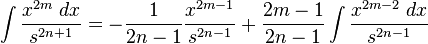# Integrating a quadratic funtion raised to m/n

I couldn't figure out what to do with this type of integration $$\int (a x^{2}+ bx +c) ^{\frac{m}{n}}dx$$ here m, n are integer numbers. Integration limit -∞ to +∞.
Will Binomial expansion work?
Please give me some clue.

## Answers and Replies

What makes you think that these integrals converge? The function to be integrated does not even converge to zero as x-> +/- infinity...!

Actually I had to solve an integral $$\int(z^{2} -8z + 36)^\frac{-3}{2} dz$$ limit - infinity to + infinity in a physics problem. dz is the differential length of a conducting wire extending from -infinity to + infinity.
Can you plz help me how can I solve above integral?

Well, the easiest way is probably to look it up it an table of integralsWikipedia states thatwhere s is the square root of x^2-a^2.....taking m=0, n=1 should help you.

maybe that is not the bsst way to go.

I rather suggest the following; use simple substitutions to reduce the integrand to
$$(x^2+1)^{-3/2}$$

then use $x=\sinh(z)$ to obtain
$$\int_{\mathbb{R}}{\frac{dz}{\cosh(z)^2}}$$

Once you have that, we can think about the next stepsLast edited:
you gave me a good idea to look it up in integral table. I found one http://www.integral-table.com/

I found quite similar integral in no. 40 in the table's list ( sorry it's quite difficult to write that integral along with its result here) . But How can I obtain that result?

have you simplified your integral to something which looks similar to
$$\int_{\mathbb{R}}{\frac{dz}{\cosh(z)^2}}$$

?

I'm puzzled at the substitution method u suggested. 1st would I let,
z^2- 8 z + 36 = u
2z -8 =du/dz
but dz = du/ (2z-8)
see there's a 'z' in the denominator on right hand side. So, letting z^2- 8 z + 36 = u won't work. What I can do now?

Well....x^2-8x+36 = (x-4)^2+20

thus your first substitution would be

y = x-4.

then

w = y/sqrt(20)

and finally

sinh(z)= w.

yeah. Thank u so much . Now I think I can manage the rest of the things.